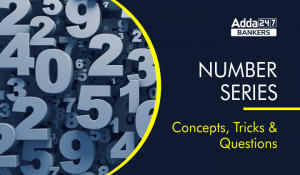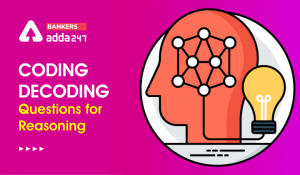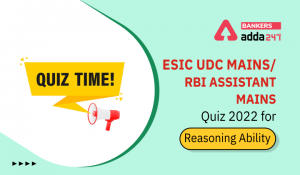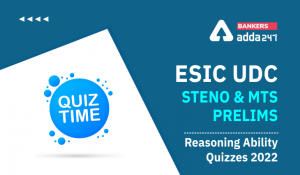Latest Banking jobs   »   Alphanumeric series

# Alphanumeric Series in Reasoning For Competitive Exams

Alphanumeric Series: Alphanumeric series is one of the most important topics of the reasoning section which is most commonly asked in the preliminary examination of banking exams. Alphanumeric series is one of the easiest topics in the reasoning section which 99% of the candidates attempt in the examination. A set of 5 questions is usually seen from the Alphanumeric series but the thing which matters the most is how quickly you can solve these questions. In this article, we are going to give you Alphanumeric series reasoning tricks and questions.

## Alphanumeric Series: Meaning

Alphanumeric series is a series that has the combination of both alphabets and numbers. To solve the alphanumeric series, candidates will be required to crack the logic behind the series.

## Alphanumeric Series: Types With Tricks

Study the following letter-number-symbol sequence and answer the questions following it

P % R 1 5 H * M T E 3 B \$ V N 4 K A 8 W I 6 2 G # U H 7 . J Q 9 L Y

Q.1 How many such vowels are there in the above arrangement each of which is immediately preceded by a consonant and immediately followed by a number

Preceded- It means before

Followed- It means After

In the above question you have to find this- Consonant Vowels Number

Common Mistake- Most of the candidates do this mistake in this kind of alphanumeric series is that they try to follow the sequence. But the correct method should be that you should find that character which is least in the series which is vowels here i.e. A,E,I,O,U. So the pair would be TE3, KA8, WI6

Ans Three

Q.2  Which of the following is the seventh to the right of the twentieth from the right in the above arrangement?

Concept- So when in the question you find two times right then substract the place values like here you would do 20 minus 7 and you will get 13 so now count 13th from the right end.

If you find the combination of left and right or right and left then add the place values. Now if you have a doubt that from which direction you will start counting then you have to count from the second element like if there is right left combination then you have to count from left.

Q.3 What should come in place of the question mark in the following series based on the above arrangement

5*T 3\$N K8I ? .QL

Ans 2 # H

When you have to find the missing element then try to crack the logic. In the above series there is a pattern that there is a gap of one element between the two characters given above.

Q.4 Four of the following five are alike in a certain way with regard to their position in the above arrangement. Which is the one that does not belong to that group

GUI, W64, 7J#, HMR, BVT

now when you will find the word alike in such kind of series then you must be clear in your mind that you have to find odd one out

Ans W64

Q.5 If we remove all the vowels in the series then which of the following element will be 7th from right end

M 6 * I A 7 \$ J # 5 4 B U 2 R Q D H L O @ P T E F

Concept- Some candidates make a mistake that they write series after dropping the vowels and then count the elements but you don’t have to follow this approach. Just imagine that vowels are not present in the series and then count the elements

Ans D

Q6 If words are arranged according to the alphabetical series from left to right then which word is third from the left

YOM MJK UGJ IMX KQZ

a) UGJ
b) IMX
c) KQZ
d) MJK
e) None of these

Common mistake- Due to the pressure of exam some candidates starts arranging the letters in word in alphabetical order but we have to arrange words not letters. So the simple concept in these kind of questions which candidates need to follow is the first word will be the one whose first letter comes before the others similarly you have to arrange all the words. In the above problem the first word will be IMX.

Ans MJK

## Alphanumeric Series Questions With Solution

Directions (1-5): Study the following arrangement carefully and answer the questions given below:
N 4 7 T U J K 3 % F @ © L N 5 P 1 8 U \$ E 2 D C 6 # 9 Z Q 8 Y*M A

Q1. Which of the following element is sixth to the left of the fifteenth from the left end of the given arrangement?
(a) 2
(b) #
(c) %
(d) \$
(e) None of these

S1. Ans.(c)

Q2. How many such numbers are there in the given arrangement each of which is immediately preceded by a consonant but not immediately followed by a letter?
(a) One
(b) None
(c) Three
(d) Two
(e) Four

S2. Ans.(e)
Sol. N47, K3%, C6#, P18

Q3. How many such symbols are there in the arrangement, each of which is immediately followed by a letter but not immediately preceded by a number?
(a) One
(b) Three
(c) None
(d) Two
(e) More than three

S3. Ans.(b)

Q4. If we remove all the numbers from the given arrangement, then which of the following element is 5th from the right end?
(a) Q
(b) T
(c) *
(d) #
(e) None of these

S4. Ans.(a)

Q5. Four of the following five are alike in a certain way based on their positions in the given arrangement and so from a group. Which is the one that does not belong to that group?
(a) DC6
(c) 9ZQ
(d) 5P1
(e) #MP

S5. Ans.(e)

Directions (6-10): Study the following arrangement carefully and answer the questions given below:
3 1 2 5 4 7 2 8 4 5 7 1 9 6 5 2 4 1 4 5 8 2 4 3 9 7 6 9 4 2 3

Q6. Which of the following digit will be sixth to the left of tenth from the right end?
(a) 6
(b) 9
(c) 5
(d) 2
(e) None of these

S6. Ans(d)
Sol. The 16th element from the right end = ‘2’.

Q7. How many 2’s is there in the given series which are followed by a number less than 6?
(a) One
(b) None
(c) Three
(d) Two
(e) More than three

S7. Ans(e)
Sol. There are four 2’s which are followed by number less than 6 i.e. ‘2 5, 2 4, 2 4 and 2 3’.

Q8. What will be the addition of fifth, seventh and tenth digit from the left end?
(a) 13
(b) 10
(c) 11
(d) 15
(e) None of these

S8. Ans(c)
Sol. The fifth, seventh and tenth digit from the left end are ‘4, 2 and 5′.
Hence, the sum is = (4+2+5)= 11

Q9. If all the perfect square digits are removed from the given series, then which of the following will be fifth to the right of sixth from the left end?
(a) 5
(b) 2
(c) 8
(d) 1
(e) None of these

S9. Ans (b)
Sol. After removing all the perfect square digits ‘3 2 5 7 2 8 5 7 6 5 2 5 8 2 3 7 6 2 3’
Then (6+5)= 11th digit from the left is ‘2’.

Q10. How many odd numbers are there in the given series which are immediately followed by a perfect square digit?
(a) One
(b) None
(c) Three
(d) Five
(e) Seven

S10.Ans(e)
Sol. There are seven odd digits which are followed by a perfect square number- ‘3 1, 5 4, 7 1, 1 9, 1 4, 3 9, 9 4’’

Directions (11-15): These questions are based on the following alphabet and digit series.
D 5 P K L 8 Z M C I 6 O Q U E 1 T N R 4 G B A 9 F S W 2 H J V 7 Y X 3

Q11. How many digits are there in above arrangement which is immediately followed by vowel?
(a) Two
(b) Four
(c) One
(d) Three
(e) None

S11. Ans(c)
Sol. ‘6 O’

Q12. If all the vowels are deleted from the above arrangement, then which of the following letter/digit is 10th to left of 5th from the right end?
(a) R
(b) 4
(c) G
(d) 9
(e) None of these

S12. Ans(b)
Sol. D 5 P K L 8 Z M C 6 Q 1 T N R 4 G B 9 F S W 2 H J V 7 Y X 3
10th +5th = 15th from right = 4

Q13. Which of the following letter/digit is 7th to the right of 13th from the right end?
(a) A
(b) 2
(c) H
(d) 9
(e) None of these

S13. Ans(e)
Sol. 13th -7th = 6th from right = J

Q14. How many consonants are there in above arrangement which is immediately preceded by consonant and immediately followed by vowel?
(a) Four
(b) Three
(c) Two
(d) One
(e) None

S14. Ans(c)
Sol. ‘M C I’, ‘G B A’

Q15. Which of the following element is exactly between ‘B’ and ‘2’ in the given arrangement?
(a) 9
(b) F
(c) S
(d) W
(e) None of these

S15. Ans(b)

## FAQs: Alphanumeric Series Reasoning Tricks

Q.1 What is alphanumeric series in reasoning?
Ans Alphanumeric series is a series that has the combination of both alphabets and numbers.

Q.2 Is there any kind of trick to solve the alphanumeric series questions?
Ans The trick to solve the alphanumeric series questions is given in the above article

Q.3 Where will I find alphanumeric questions for bank exams?
Ans The alphanumeric questions for bank exams is given in the above article and if you want to practice more questions then you can download add247 app where you will find quizzes for bank exams free of cost.

Current Affairs#### Congratulations!Incorrect details? Fill the form again here

•Number Series Key Concept, Short Tricks,...
•Coding-Decoding Reasoning, Short Tricks,...
•Time & Distance Formula, Tricks, Que...
•Reasoning Ability Quiz For RBI Assistant...
•Reasoning Ability Quiz For ESIC- UDC, St...
•Reasoning Ability Quiz For ESIC- UDC, St...